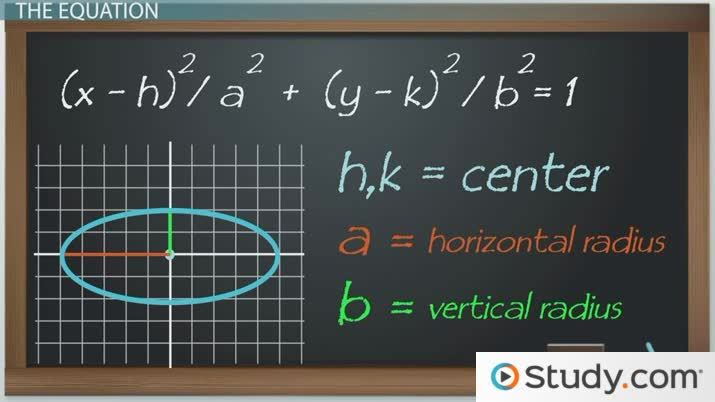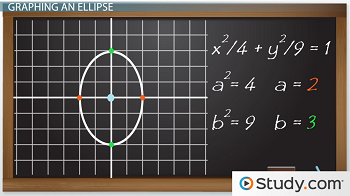# Defining and Graphing Ellipses in Algebra

Lesson Transcript
Instructor: Yuanxin (Amy) Yang Alcocer

Amy has a master's degree in secondary education and has been teaching math for over 9 years. Amy has worked with students at all levels from those with special needs to those that are gifted.

An ellipse is a circle that has been stretched, with the horizontal path from the radius shorter than the vertical. Learn how to define the ellipse, and how the equation is used in the graphing of ellipses in algebra. Updated: 10/04/2021

## An Ellipse

An ellipse can be described as a stretched-out circle. I picture two people pulling at either end of a circle and stretching it out. Or you can picture two dogs playing tug-of-war with a circular disk. Any which way, ellipses are important to learn in math because they are also a conic section, meaning that you can get an ellipse by slicing a cone. Slice an ice cream waffle cone at an angle and you will have an ellipse.An error occurred trying to load this video.

Try refreshing the page, or contact customer support.

Coming up next: How to Write the Equation of an Ellipse in Standard Form

### You're on a roll. Keep up the good work!

Replay
Your next lesson will play in 10 seconds
• 0:33 The Equation
• 1:30 Graphing an Ellipse
• 2:38 Graphing Another
• 3:50 Lesson Summary
Save Save

Want to watch this again later?

Timeline
Autoplay
Autoplay
Speed Speed

## The Equation

Just like with circles, an ellipse has a center. Unlike circles, an ellipse has two different measurements for its radius, which is the distance from the edge to the center. Notice how an ellipse is longer in one direction than the other? Because of this, an ellipse will have a radius measurement for one direction and another radius measurement for the other direction. Our equation of an ellipse takes into account all of these measurements. Our equation is (x - h)^2 / a^2 + (y - k)^2 / b^2 = 1, where (h, k) is the center of our ellipse, a is the radius in the horizontal direction, and b is the radius in the vertical direction. Notice that there is always a plus in between the x and y parts of the equation and that the equation always equals 1.

## Graphing an Ellipse

Let's see how we can use this equation to help us graph an ellipse. Say we want to graph the equation x^2 / 4 + y^2 / 9 = 1. First, we notice that the center of our ellipse is located at (0, 0) since there are no numbers being subtracted or added to our x or y. Next, we figure out how far out our ellipse edges will go. Our a^2 is 4, so that means my a is 2. If my a is 2, then that means my ellipse goes out 2 spaces to the left and 2 spaces to the right of my center. I can go ahead and draw two dots at those two places. Next, I see that my b^2 is 9, which means my b is 3. Since my b is 3, then my other two dots will be drawn 3 spaces above my center and 3 spaces below my center. Now that I have my dots, I can go and draw my ellipse - my stretched-out circle - making sure to touch all those dots.To unlock this lesson you must be a Study.com Member.

### Register to view this lesson

Are you a student or a teacher?